# Electronics and Communication Engineering - Analog Electronics

6.

A 120 V, 30 Hz source feeds a half wave rectifier circuit through a 4 : 1 step down transformer, the average output voltage is

 A. 30 V B. 13.5 V C. 10 V D. 7.07 V

Explanation: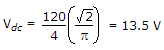.

7.

The slew rate of an ideal op-amp is

 A. very slow B. slow C. fast D. infinitely fast

Explanation:

Slew rate signifies the fastest change which can occurs in output voltage. In an ideal op-amp the output can change instantaneously.

8.

Regulation of the DC power supply of 12 V, 100 mA is the effective resistance of power supply is 20 Ω

 A. 22% B. 131% C. 30% D. 20%

Explanation: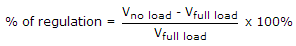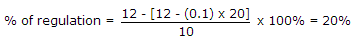.

9.

A bridge diode circuit using ideal diodes has in input voltage of 20 sin ωt volts. The average and rms values of output voltage are

 A.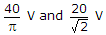B.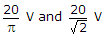C.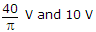D.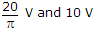Explanation: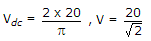.

10.

In the circuit of figure v1 = 8 V and v2 = 0. Which diode will conduct (Assume ideal diodes)?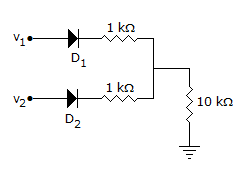A. D1 only B. D2 only C. Both D1 and D2 D. Neither D1 nor D2